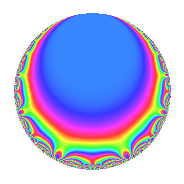Properties

 Label 8016.2.a.kLevel 8016 Weight 2 Character orbit 8016.a Self dual Yes Analytic conductor 64.008 Analytic rank 0 Dimension 2 CM No Inner twists 1

Related objects

Newspace parameters

 Level: $$N$$ = $$8016 = 2^{4} \cdot 3 \cdot 167$$ Weight: $$k$$ = $$2$$ Character orbit: $$[\chi]$$ = 8016.a (trivial)

Newform invariants

 Self dual: Yes Analytic conductor: $$64.0080822603$$ Analytic rank: $$0$$ Dimension: $$2$$ Coefficient field: $$\Q(\sqrt{2})$$ Coefficient ring: $$\Z[a_1, \ldots, a_{5}]$$ Coefficient ring index: $$1$$ Fricke sign: $$-1$$ Sato-Tate group: $\mathrm{SU}(2)$

$q$-expansion

Coefficients of the $$q$$-expansion are expressed in terms of $$\beta = \sqrt{2}$$. We also show the integral $$q$$-expansion of the trace form.

 $$f(q)$$ $$=$$ $$q$$ $$+ q^{3}$$ $$+ ( -2 + \beta ) q^{5}$$ $$+ 2 \beta q^{7}$$ $$+ q^{9}$$ $$+O(q^{10})$$ $$q$$ $$+ q^{3}$$ $$+ ( -2 + \beta ) q^{5}$$ $$+ 2 \beta q^{7}$$ $$+ q^{9}$$ $$-2 q^{11}$$ $$+ 2 \beta q^{13}$$ $$+ ( -2 + \beta ) q^{15}$$ $$+ ( -4 + \beta ) q^{17}$$ $$+ ( -4 - 2 \beta ) q^{19}$$ $$+ 2 \beta q^{21}$$ $$+ 4 \beta q^{23}$$ $$+ ( 1 - 4 \beta ) q^{25}$$ $$+ q^{27}$$ $$+ 2 q^{29}$$ $$+ ( -4 + 2 \beta ) q^{31}$$ $$-2 q^{33}$$ $$+ ( 4 - 4 \beta ) q^{35}$$ $$-4 q^{37}$$ $$+ 2 \beta q^{39}$$ $$+ ( -4 + \beta ) q^{41}$$ $$+ ( 4 + 3 \beta ) q^{43}$$ $$+ ( -2 + \beta ) q^{45}$$ $$+ ( 10 - 2 \beta ) q^{47}$$ $$+ q^{49}$$ $$+ ( -4 + \beta ) q^{51}$$ $$+ ( -2 + 3 \beta ) q^{53}$$ $$+ ( 4 - 2 \beta ) q^{55}$$ $$+ ( -4 - 2 \beta ) q^{57}$$ $$+ ( 10 + 2 \beta ) q^{59}$$ $$+ 10 \beta q^{61}$$ $$+ 2 \beta q^{63}$$ $$+ ( 4 - 4 \beta ) q^{65}$$ $$-\beta q^{67}$$ $$+ 4 \beta q^{69}$$ $$+ ( 4 + 6 \beta ) q^{71}$$ $$+ ( -2 + 10 \beta ) q^{73}$$ $$+ ( 1 - 4 \beta ) q^{75}$$ $$-4 \beta q^{77}$$ $$+ ( 6 + 3 \beta ) q^{79}$$ $$+ q^{81}$$ $$+ ( 6 + 4 \beta ) q^{83}$$ $$+ ( 10 - 6 \beta ) q^{85}$$ $$+ 2 q^{87}$$ $$+ ( -2 - 8 \beta ) q^{89}$$ $$+ 8 q^{91}$$ $$+ ( -4 + 2 \beta ) q^{93}$$ $$+ 4 q^{95}$$ $$+ ( -6 - 8 \beta ) q^{97}$$ $$-2 q^{99}$$ $$+O(q^{100})$$ $$\operatorname{Tr}(f)(q)$$ $$=$$ $$2q$$ $$\mathstrut +\mathstrut 2q^{3}$$ $$\mathstrut -\mathstrut 4q^{5}$$ $$\mathstrut +\mathstrut 2q^{9}$$ $$\mathstrut +\mathstrut O(q^{10})$$ $$2q$$ $$\mathstrut +\mathstrut 2q^{3}$$ $$\mathstrut -\mathstrut 4q^{5}$$ $$\mathstrut +\mathstrut 2q^{9}$$ $$\mathstrut -\mathstrut 4q^{11}$$ $$\mathstrut -\mathstrut 4q^{15}$$ $$\mathstrut -\mathstrut 8q^{17}$$ $$\mathstrut -\mathstrut 8q^{19}$$ $$\mathstrut +\mathstrut 2q^{25}$$ $$\mathstrut +\mathstrut 2q^{27}$$ $$\mathstrut +\mathstrut 4q^{29}$$ $$\mathstrut -\mathstrut 8q^{31}$$ $$\mathstrut -\mathstrut 4q^{33}$$ $$\mathstrut +\mathstrut 8q^{35}$$ $$\mathstrut -\mathstrut 8q^{37}$$ $$\mathstrut -\mathstrut 8q^{41}$$ $$\mathstrut +\mathstrut 8q^{43}$$ $$\mathstrut -\mathstrut 4q^{45}$$ $$\mathstrut +\mathstrut 20q^{47}$$ $$\mathstrut +\mathstrut 2q^{49}$$ $$\mathstrut -\mathstrut 8q^{51}$$ $$\mathstrut -\mathstrut 4q^{53}$$ $$\mathstrut +\mathstrut 8q^{55}$$ $$\mathstrut -\mathstrut 8q^{57}$$ $$\mathstrut +\mathstrut 20q^{59}$$ $$\mathstrut +\mathstrut 8q^{65}$$ $$\mathstrut +\mathstrut 8q^{71}$$ $$\mathstrut -\mathstrut 4q^{73}$$ $$\mathstrut +\mathstrut 2q^{75}$$ $$\mathstrut +\mathstrut 12q^{79}$$ $$\mathstrut +\mathstrut 2q^{81}$$ $$\mathstrut +\mathstrut 12q^{83}$$ $$\mathstrut +\mathstrut 20q^{85}$$ $$\mathstrut +\mathstrut 4q^{87}$$ $$\mathstrut -\mathstrut 4q^{89}$$ $$\mathstrut +\mathstrut 16q^{91}$$ $$\mathstrut -\mathstrut 8q^{93}$$ $$\mathstrut +\mathstrut 8q^{95}$$ $$\mathstrut -\mathstrut 12q^{97}$$ $$\mathstrut -\mathstrut 4q^{99}$$ $$\mathstrut +\mathstrut O(q^{100})$$

Embeddings

For each embedding $$\iota_m$$ of the coefficient field, the values $$\iota_m(a_n)$$ are shown below.

For more information on an embedded modular form you can click on its label.

Label $$\iota_m(\nu)$$ $$a_{2}$$ $$a_{3}$$ $$a_{4}$$ $$a_{5}$$ $$a_{6}$$ $$a_{7}$$ $$a_{8}$$ $$a_{9}$$ $$a_{10}$$
1.1
 −1.41421 1.41421
0 1.00000 0 −3.41421 0 −2.82843 0 1.00000 0
1.2 0 1.00000 0 −0.585786 0 2.82843 0 1.00000 0
 $$n$$: e.g. 2-40 or 990-1000 Significant digits: Format: Complex embeddings Normalized embeddings Satake parameters Satake angles

Inner twists

This newform does not admit any (nontrivial) inner twists.

Atkin-Lehner signs

$$p$$ Sign
$$2$$ $$-1$$
$$3$$ $$-1$$
$$167$$ $$-1$$

Hecke kernels

This newform can be constructed as the intersection of the kernels of the following linear operators acting on $$S_{2}^{\mathrm{new}}(\Gamma_0(8016))$$:

 $$T_{5}^{2}$$ $$\mathstrut +\mathstrut 4 T_{5}$$ $$\mathstrut +\mathstrut 2$$ $$T_{7}^{2}$$ $$\mathstrut -\mathstrut 8$$ $$T_{11}$$ $$\mathstrut +\mathstrut 2$$ $$T_{13}^{2}$$ $$\mathstrut -\mathstrut 8$$